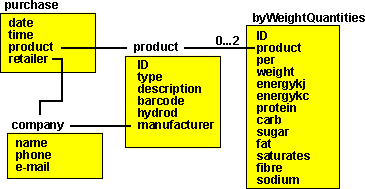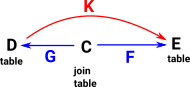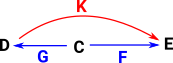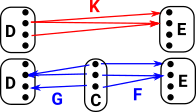# Maths - Category Theory - Kan Extensions

### Kan Extensions

Left Kan Extension Right Kan ExtensionGiven functors F and K the left Kan extension is a functor which closes the triangle and is unique in that it has a map to every other functor from D to E which makes the triangle commute. That is, every other functor factors through it. Given functors F and K the right Kan extension is a functor which closes the triangle and is unique in that it has a map from every other functor from D to E which makes the triangle commute. That is, every other functor factors through it.

## Examples

### Example - Relational Database Join Tables

 A relational database consists of tables.If we want a many to many relationship (multiple records in a table are associated with multiple records in another table) we need to add a join table.### Example - Generalise Maps in Sets

 Here D,C and E are sets. The arrows G and F are ordinary mappings between sets (that is they may be injections, surjections or bijections). This allows us to generate an arrow K which may be a more general type of arrow. To get some intuition what this means it helps to look inside these sets and functions.In this way we can construct a new type of category with mappings where elements in the domain may map to zero or more than one elements in the codomain.### An Example Natural Numbers to Integers

 Here D,C and E are natural numbers. The arrows G and F are additions of natural numbers. This allows us to generate an arrow K which may be a more general type of arrow which has now generated the negative numbers and so created the integers.If we start off with natural numbers (0,1,2,3...) and we define an operation (plus) on it, by taking the inverse of this operation (subtraction) we generate the negative numbers which gives us the integers.

It is often the case that taking the inverse will generate new objects. Another example would be taking the inverse of multiplication to give rational numbers.

However we can generalise even further than inverse operations to Kan extensions.

 Consider natural numbers. We can include the negative numbers but how can we extend addition so that it has the correct properties for negative numbers?## Computer Code

How can we write code to implement this?

```data LKan C D E where
LKan : (C b -> E) -> D b -> LKan C D E```

## Another Way to look at it

 K is a functor which injects I into A D is a functor which then injects this into C### AGDA Code

```{-# OPTIONS --universe-polymorphism #-}
module Categories.Lan where

open import Level
open import Categories.Category
open import Categories.Functor hiding (_≡_)
open import Categories.NaturalTransformation

record Lan {o₀ ℓ₀ e₀} {o₁ ℓ₁ e₁} {o₂ ℓ₂ e₂}
{A : Category o₀ ℓ₀ e₀} {B : Category o₁ ℓ₁ e₁} {C : Category o₂ ℓ₂ e₂}
(F : Functor A B) (X : Functor A C) : Set (o₀ ⊔ ℓ₀ ⊔ e₀ ⊔ o₁ ⊔ ℓ₁ ⊔ e₁ ⊔ o₂ ⊔ ℓ₂ ⊔ e₂) where
field
L : Functor B C
ε : NaturalTransformation X (L ∘ F)

σ : (M : Functor B C) -> (α : NaturalTransformation X (M ∘ F)) -> NaturalTransformation L M

.σ-unique : {M : Functor B C} -> {α : NaturalTransformation X (M ∘ F)} ->
(σ′ : NaturalTransformation L M) -> α ≡ (σ′ ∘ʳ F) ∘₁ ε -> σ′ ≡ σ M α
.commutes : (M : Functor B C) -> (α : NaturalTransformation X (M ∘ F)) -> α ≡ (σ M α ∘ʳ F) ∘₁ ε```

### Ends

An 'end' is a generalsation of a product.

forall a . p a a

see Bartosz Milewski on Youtube here

### Comma and Slice Categories

Also related are comma and slice category.

### Computer Code

 There exists b such that: ```data LKan g h a where LKan : (g b -> a) -> h b -> LKan g h a```

where

• (g b -> a) as an object of the comma category Ka
• f b as the mapping of b under the functor F
• b - the projection of this object back to C

where

Signature Logic Contains
A+B   an element of A or an element of B
(A,B) or A*B   tuple (an element of both A and B)
A -> BAB an element of B for every element of A
A -> B -> C -> DA,B,CD
A -> (B -> (C -> D))   same as A -> B -> C -> D
(A, B, C) -> D   same as A -> B -> C -> D (uncurried)
(A -> B) -> C(A->B)C like a second level of forall on A

### Kan Extensions on Other Sites

A Haskell implementation of Kan extensions by Edward Kmett

explained by Bartosz Milewski here.

Kan extensions on the page about cubical type theory here.

Where I can, I have put links to Amazon for books that are relevant to the subject, click on the appropriate country flag to get more details of the book or to buy it from them.The Princeton Companion to Mathematics - This is a big book that attempts to give a wide overview of the whole of mathematics, inevitably there are many things missing, but it gives a good insight into the history, concepts, branches, theorems and wider perspective of mathematics. It is well written and, if you are interested in maths, this is the type of book where you can open a page at random and find something interesting to read. To some extent it can be used as a reference book, although it doesn't have tables of formula for trig functions and so on, but where it is most useful is when you want to read about various topics to find out which topics are interesting and relevant to you.